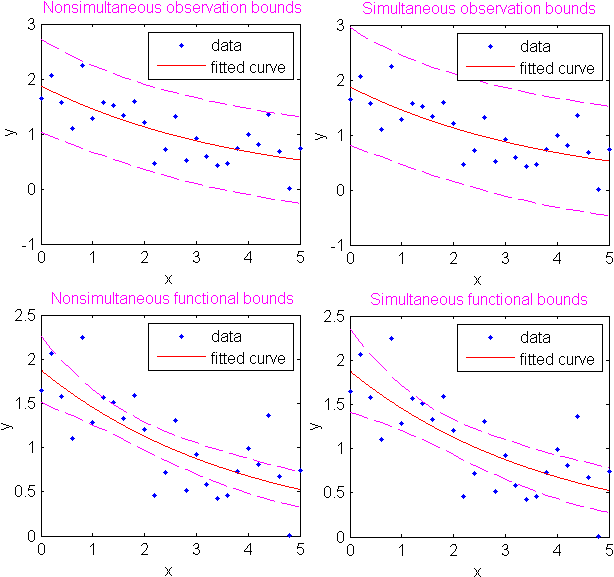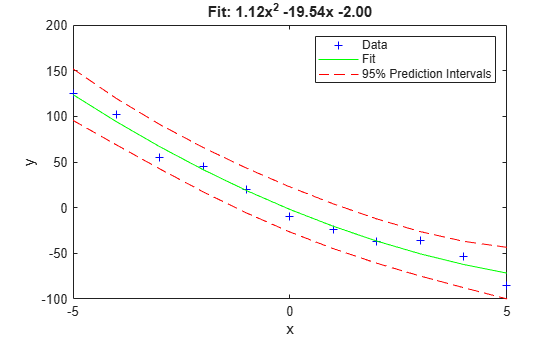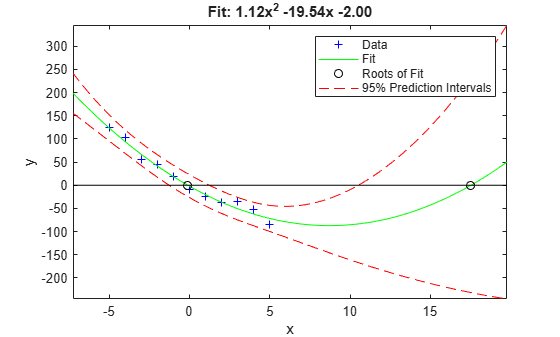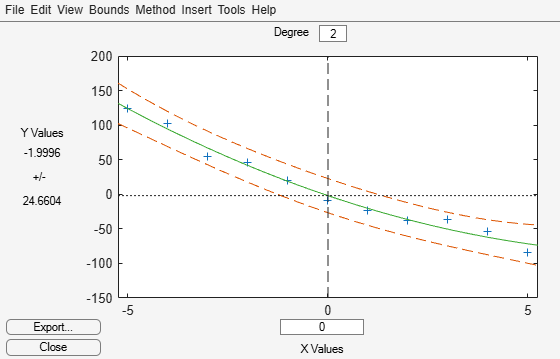Main Content

# polyconf

Polynomial confidence intervals

## Syntax

```Y = polyconf(p,X) [Y,DELTA] = polyconf(p,X,S) [Y,DELTA] = polyconf(p,X,S,param1,val1,param2,val2,...) ```

## Description

`Y = polyconf(p,X)` evaluates the polynomial `p` at the values in `X`. `p` is a vector of coefficients in descending powers.

`[Y,DELTA] = polyconf(p,X,S)` takes outputs `p` and `S` from `polyfit` and generates 95% prediction intervals `Y ± DELTA` for new observations at the values in `X`.

`[Y,DELTA] = polyconf(p,X,S,param1,val1,param2,val2,...)` specifies optional parameter name/value pairs chosen from the following list.

ParameterValue
`'alpha'`

A value between 0 and 1 specifying a confidence level of `100*(1-alpha)`%. The default is `0.05`.

`'mu'`

A two-element vector containing centering and scaling parameters. With this option, `polyconf` uses `(X-mu(1))/mu(2)` in place of `X`.

`'predopt'`

Either `'observation'` (the default) to compute prediction intervals for new observations at the values in `X`, or `'curve'` to compute confidence intervals for the fit evaluated at the values in `X`. See below.

`'simopt'`

Either `'off'` (the default) for nonsimultaneous bounds, or `'on'` for simultaneous bounds. See below.

The `'predopt'` and `'simopt'` parameters can be understood in terms of the following functions:

• p(x) — the unknown mean function estimated by the fit

• l(x) — the lower confidence bound

• u(x) — the upper confidence bound

Suppose you make a new observation yn+1 at xn+1, so that

yn+1(xn+1) = p(xn+1) + εn+1

By default, the interval [ln+1(xn+1), un+1(xn+1)] is a 95% confidence bound on yn+1(xn+1).

The following combinations of the `'predopt'` and `'simopt'` parameters allow you to specify other bounds.

`'simopt'``'predopt'`Bounded Quantity
`'off'``'observation'`

yn+1(xn+1) (default)

`'off'``'curve'`

p(xn+1)

`'on'``'observation'`

yn+1(x), for all x

`'on'``'curve'`

p(x), for all x

In general, `'observation'` intervals are wider than `'curve'` intervals, because of the additional uncertainty of predicting a new response value (the curve plus random errors). Likewise, simultaneous intervals are wider than nonsimultaneous intervals, because of the additional uncertainty of bounding values for all predictors x.## Examples

collapse all

Fit a polynomial to a sample data set, and estimate the 95% prediction intervals and the roots of the fitted polynomial. Plot the data and the estimations, and display the fitted polynomial expression using the helper function `polystr`, whose code appears at the end of this example.

Generate sample data points `(x,y)` with a quadratic trend.

```rng('default') % For reproducibility x = -5:5; y = x.^2 - 20*x - 3 + 5*randn(size(x));```

Fit a second degree polynomial to the data by using `polyfit`.

```degree = 2; % Degree of the fit [p,S] = polyfit(x,y,degree);```

Estimate the 95% prediction intervals by using `polyconf`.

```alpha = 0.05; % Significance level [yfit,delta] = polyconf(p,x,S,'alpha',alpha);```

Plot the data, fitted polynomial, and prediction intervals. Display the fitted polynomial expression using the helper function `polystr`.

```plot(x,y,'b+') hold on plot(x,yfit,'g-') plot(x,yfit-delta,'r--',x,yfit+delta,'r--') legend('Data','Fit','95% Prediction Intervals') title(['Fit: ',texlabel(polystr(round(p,2)))]) hold off```Find the roots of the polynomial `p`.

`r = roots(p)`
```r = 2×1 17.5152 -0.1017 ```

Because the roots are real values, you can plot them as well. Estimate the fitted values and prediction intervals for the x interval that includes the roots. Then, plot the roots and the estimations.

```if isreal(r) xmin = min([r(:);x(:)]); xrange = range([r(:);x(:)]); xExtended = linspace(xmin - 0.1*xrange, xmin + 1.1*xrange,1000); [yfitExtended,deltaExtended] = polyconf(p,xExtended,S,'alpha',alpha); plot(x,y,'b+') hold on plot(xExtended,yfitExtended,'g-') plot(r,zeros(size(r)),'ko') plot(xExtended,yfitExtended-deltaExtended,'r--') plot(xExtended,yfitExtended+deltaExtended,'r--') plot(xExtended,zeros(size(xExtended)),'k-') legend('Data','Fit','Roots of Fit','95% Prediction Intervals') title(['Fit: ',texlabel(polystr(round(p,2)))]) axis tight hold off end```Alternatively, you can use `polytool` for interactive polynomial fitting.

`polytool(x,y,degree,alpha)`Helper Function

The `polystr.m` file defines the `polystr` helper function.

`type polystr.m % Display contents of polystr.m file`
```function s = polystr(p) % POLYSTR Converts a vector of polynomial coefficients to a character string. % S is the string representation of P. if all(p == 0) % All coefficients are 0. s = '0'; else d = length(p) - 1; % Degree of polynomial. s = []; % Initialize s. for a = p if a ~= 0 % Coefficient is nonzero. if ~isempty(s) % String is not empty. if a > 0 s = [s ' + ']; % Add next term. else s = [s ' - ']; % Subtract next term. a = -a; % Value to subtract. end end if a ~= 1 || d == 0 % Add coefficient if it is ~=1 or polynomial is constant. s = [s num2str(a)]; if d > 0 % For nonconstant polynomials, add *. s = [s '*']; end end if d >= 2 % For terms of degree > 1, add power of x. s = [s 'x^' int2str(d)]; elseif d == 1 % No power on x term. s = [s 'x']; end end d = d - 1; % Increment loop: Add term of next lowest degree. end end end ```

## See Also

Introduced before R2006a

Download ebook